# Trawl processes

Here we describe a non-parametric estimation method for the trawl function which has been proposed in the article by (Sauri and Veraart 2022).

Let $$L$$ be a homogeneous Lévy basis on $$\mathbb{R}^{2}$$ with characteristic triplet $$(\gamma,b,\nu)$$, and let $$a:\mathbb{R}^{+}\rightarrow\mathbb{R}^{+}$$ be a non-increasing integrable function and put $A=\left\{ (r,y):r\leq0,0\leq y\leq a(-r)\right\} .$ We define a trawl process by $X_{t}:=L(A_{t}),$ where $$A_{t}:=A+(t,0)$$. I.e. we can represent $$X$$ as $X_t =\int_{(-\infty,t]\times \mathbb{R}} \mathbb{I}_{(0, a(t-s))}(x)L(dx,ds), \mbox{ for } t \ge 0.$

# Non-parametric estimation of the trawl function

We implement the estimator of the function $$a$$ proposed in (Sauri and Veraart 2022).

We consider $$n\in\mathbb{N}$$ equidistant observations of $$X$$, denoted by $$(X_{i\Delta_{n}})_{i=0}^{n-1}$$. We set
$\hat{\varGamma}_{l}:=\frac{1}{n}\sum_{k=0}^{n-1-l}(X_{(l+k)\Delta_{n}}-\bar{X}_{n})(X_{k\Delta_{n}}-\bar{X}_{n}),\,\,\,l=0,\ldots,n-1,$ where $$\bar{X}_{n}=\frac{1}{n}\sum_{k=1}^{n}X_{(k-1)\Delta_{n}}$$.

The estimator of the trawl function $$a(t)$$, for $$t>0$$ is given by
$\hat{a}(t) =-\Delta_{n}^{-1}\left[\hat{\varGamma}_{l+1}-\hat{\varGamma}_{l}\right],\,\,\,\text{if }\Delta_{n}l\leq t<(l+1)\Delta_{n},$ while for $$t=0$$, we set $\hat{a}(0)=\frac{1}{2\Delta_{n}n}\sum_{k=0}^{n-2}(\delta_{k}X)^{2},\,\,\,\delta_{k}X:=X_{(k+1)\Delta_{n}}-X_{k\Delta_{n}}.$ The code also provides the functionality to estimate the function $$a$$ in the case when $$t=0$$ by using the estimator used for general $$t$$, but it is typically not recommended, see the discussion in Sauri and Veraart (2022).

We now demonstrate the trawl function estimation in practice.

library(ambit)
library(ggplot2)
library(latex2exp)

First, we generate suitable data. Here, we work with a Poisson, Negative Binomial and Gaussian trawl process, each with exponential trawl function.

###Choosing the sampling scheme
my_n <- 5000
my_delta <- 0.1
my_t <- my_n*my_delta

###Choosing the model parameter
#Exponential trawl:
my_lambda <- 1
###Poisson-Exponential trawl
my_v <- 1

##Gaussian-Exponential trawl
my_mu <- 0
my_sigma <-1

#Negative binomial model

my_theta <- 0.2
my_m <- (1-my_theta)^2/my_theta

#Set the seed
set.seed(123)

Poi_data<-ambit::sim_weighted_trawl(my_n, my_delta, "Exp", my_lambda, "Poi", my_v)$path NB_data<-ambit::sim_weighted_trawl(my_n, my_delta, "Exp", my_lambda, "NegBin", c(my_m,my_theta))$path
Gau_data<-ambit::sim_weighted_trawl(my_n, my_delta, "Exp", my_lambda, "Gauss", c(my_mu,my_sigma))$path #Plot the path df1 <- base::data.frame(time = base::seq(0,my_n,1), value=Poi_data) p1 <- ggplot(df1, aes(x=time, y=Poi_data))+ geom_line()+ xlab("l")+ ylab("Poisson trawl process") p1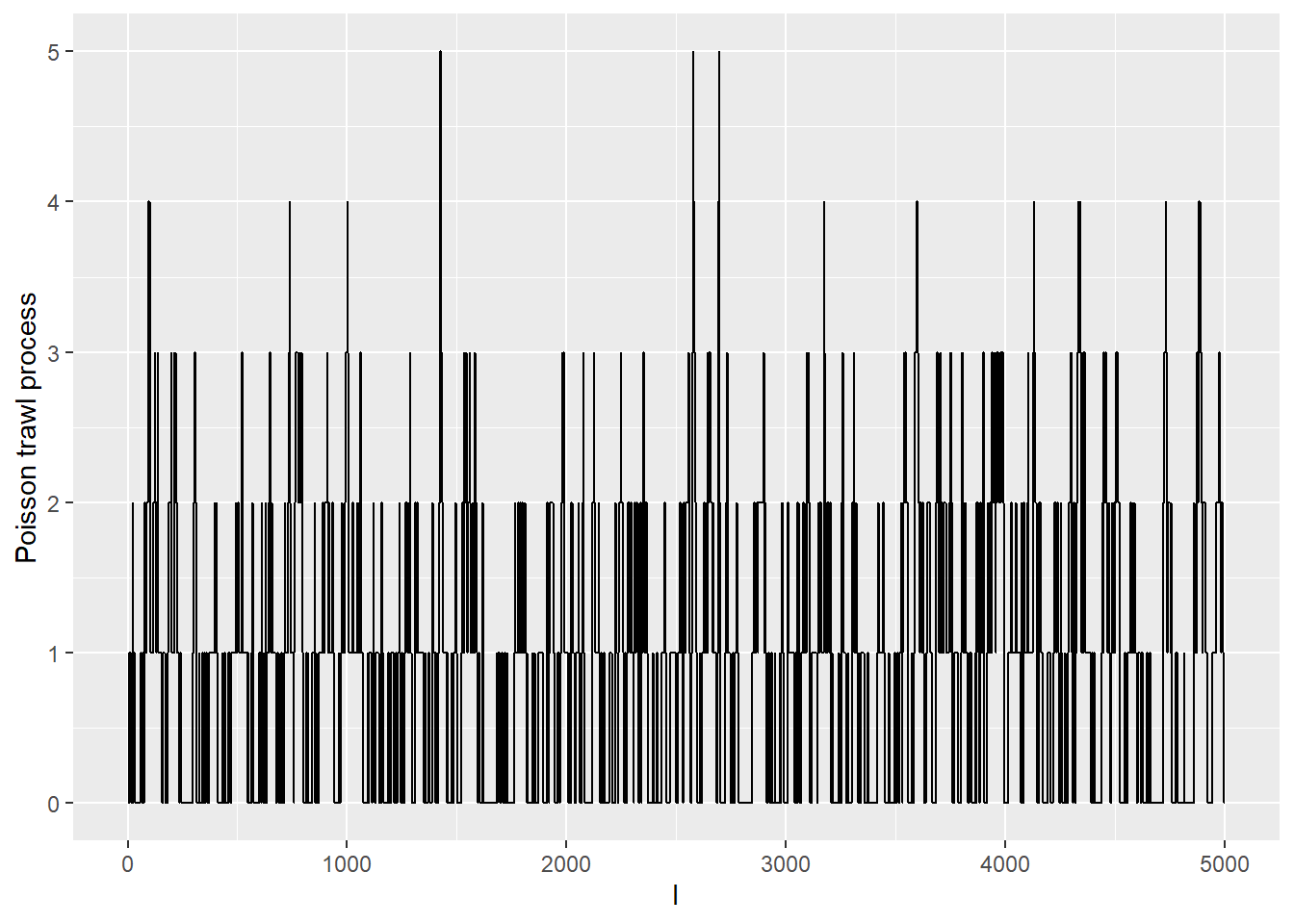df2 <- base::data.frame(time = base::seq(0,my_n,1), value=NB_data) p2 <- ggplot(df2, aes(x=time, y=NB_data))+ geom_line()+ xlab("l")+ ylab("Negative binomial trawl process") p2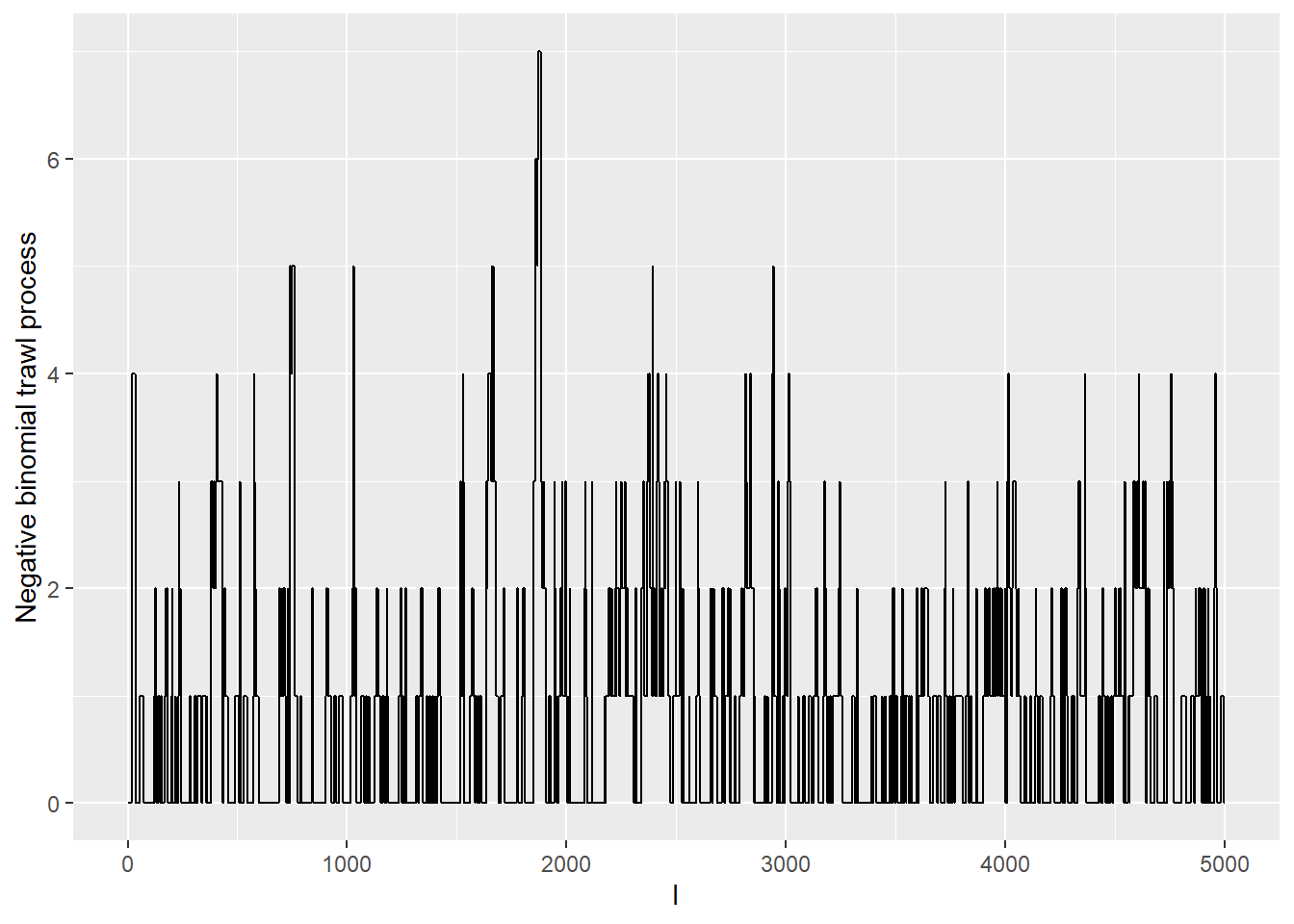df3 <- base::data.frame(time = base::seq(0,my_n,1), value=Gau_data) p3 <- ggplot(df3, aes(x=time, y=Gau_data))+ geom_line()+ xlab("l")+ ylab("Gaussian trawl process") p3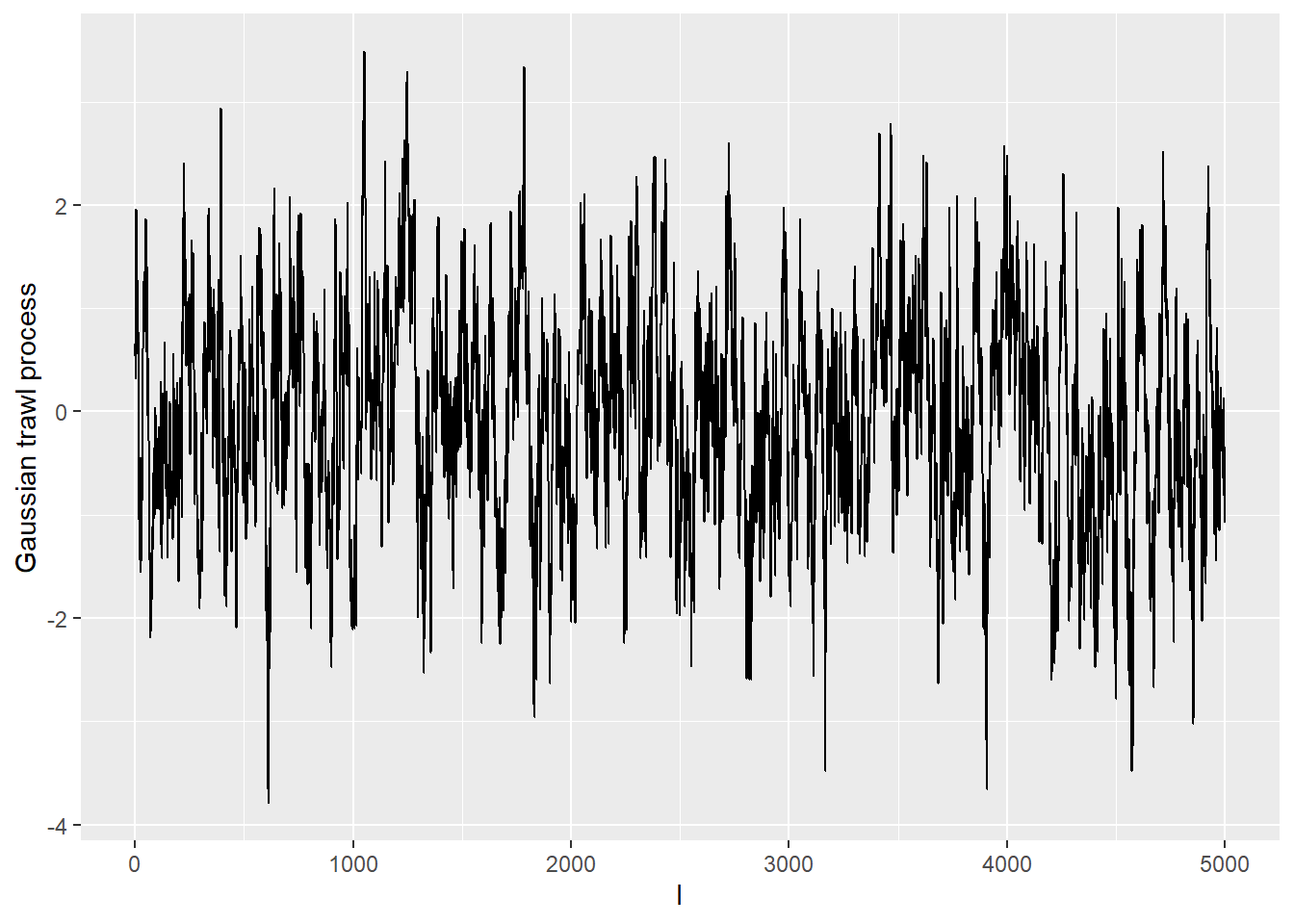We can now estimate the (exponential) trawl function nonparametrically using the nonpar_trawlest function as follows. my_lag <- 100+1 PoiEx_trawl <- nonpar_trawlest(Poi_data, my_delta, lag=my_lag)$a_hat

l_seq <- seq(from = 0,to = (my_lag-1), by = 1)
esttrawlfct.data <- data.frame(l=l_seq[1:31],
value=PoiEx_trawl[1:31])
p1 <- ggplot(esttrawlfct.data, aes(x=l,y=value))+
geom_point(size=3)+
geom_function(fun = function(x) acf_Exp(x*my_delta,my_lambda), colour="red", size=1.5)+
xlab("l")+
ylab(TeX("$\\hat{a}(\\cdot)$ for Poisson trawl process"))
p1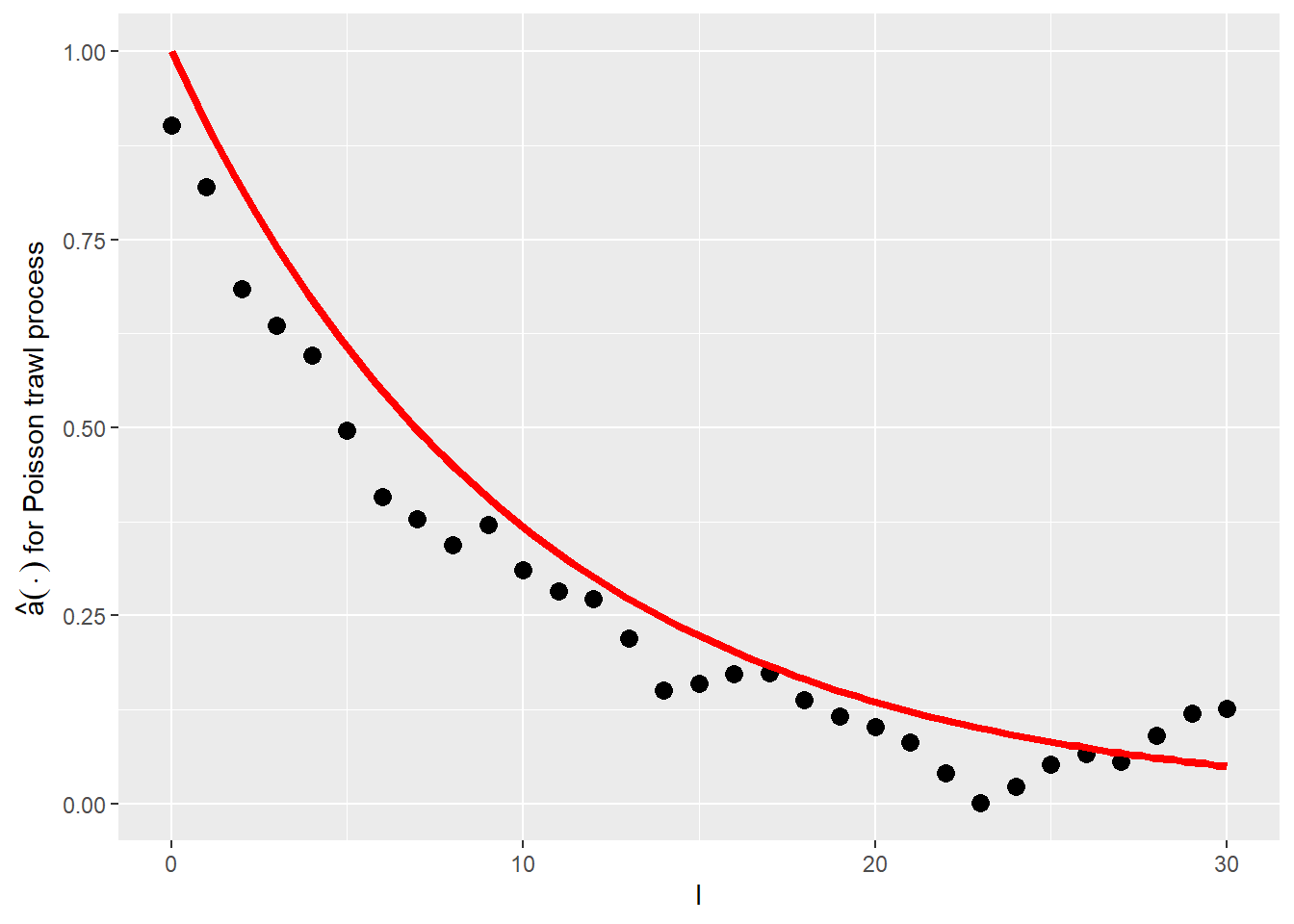my_lag <- 100+1

NBEx_trawl <- nonpar_trawlest(NB_data, my_delta, lag=my_lag)$a_hat l_seq <- seq(from = 0,to = (my_lag-1), by = 1) esttrawlfct.data <- data.frame(l=l_seq[1:31], value=NBEx_trawl[1:31]) p2 <- ggplot(esttrawlfct.data, aes(x=l,y=value))+ geom_point(size=3)+ geom_function(fun = function(x) acf_Exp(x*my_delta,my_lambda), colour="red", size=1.5)+ xlab("l")+ ylab(TeX("$\\hat{a}(\\cdot)$for NegBin trawl process")) p2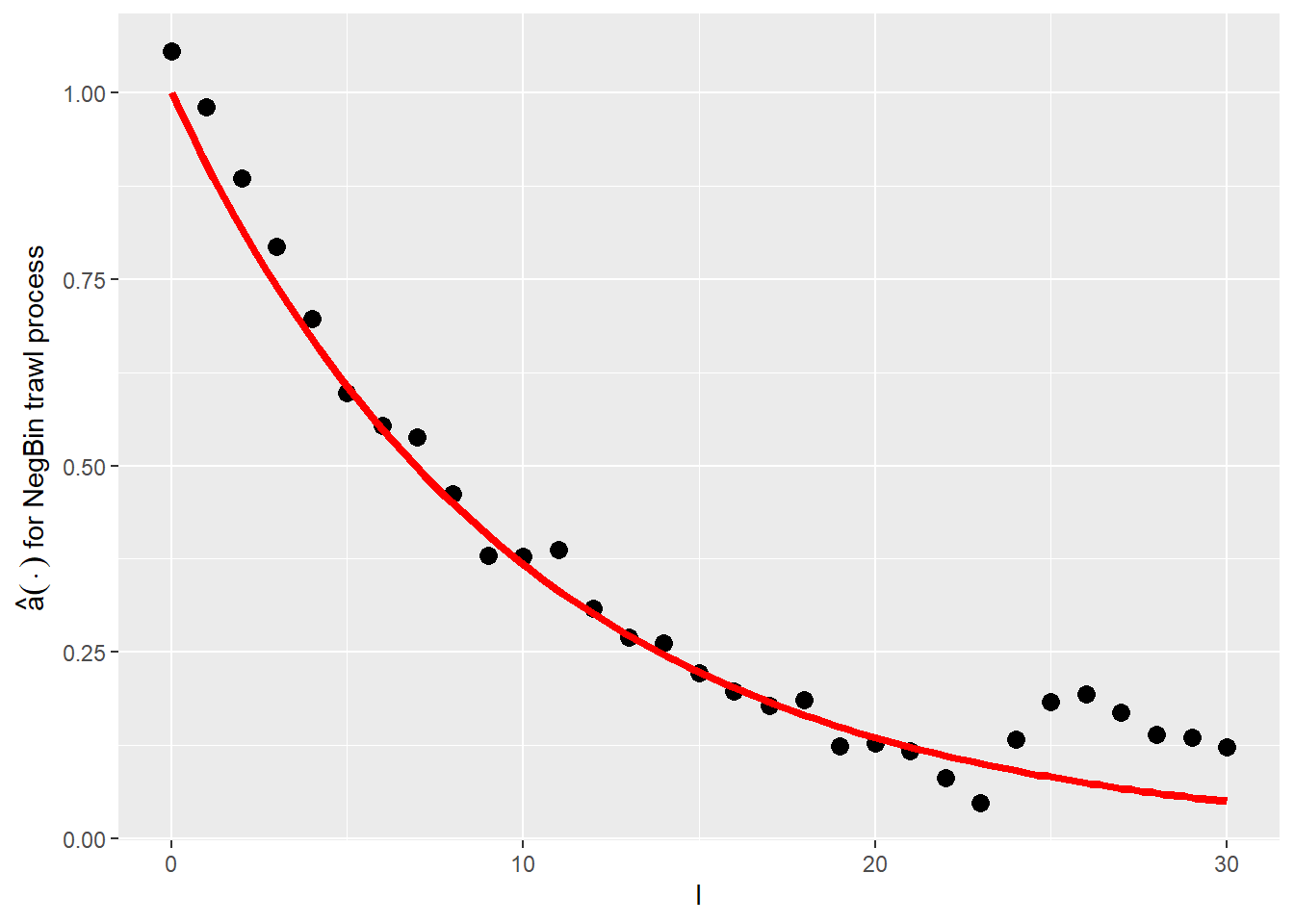my_lag <- 100+1 GaussEx_trawl <- nonpar_trawlest(Gau_data, my_delta, lag=my_lag)$a_hat

l_seq <- seq(from = 0,to = (my_lag-1), by = 1)
esttrawlfct.data <- data.frame(l=l_seq[1:31],
value=GaussEx_trawl[1:31])
p3 <- ggplot(esttrawlfct.data, aes(x=l,y=value))+
geom_point(size=3)+
geom_function(fun = function(x) acf_Exp(x*my_delta,my_lambda), colour="red", size=1.5)+
xlab("l")+
ylab(TeX("$\\hat{a}(\\cdot)$ for Gaussian trawl process"))
p3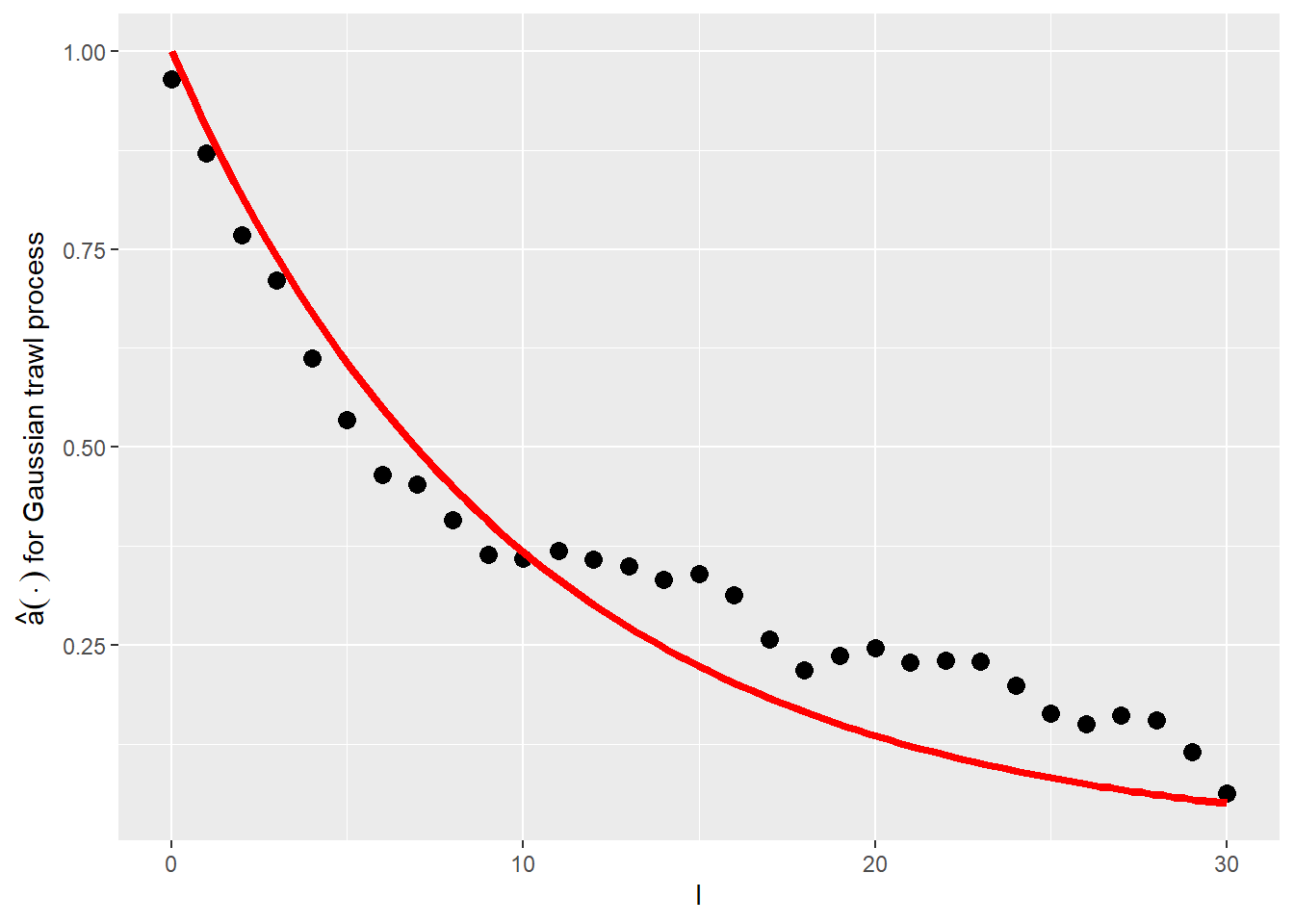Throughout, we superimposed the true trawl function used in the simulation in red. We note that the above results are only for one path. Detailed simulation results for the methodology are available in the supplementary material to (Sauri and Veraart 2022).

# Functions used for the central limit theorem

Under the technical assumptions stated in (Sauri and Veraart 2022), when $$\mu =0$$, we have the following result, for all $$t\geq 0$$, as $$n\rightarrow\infty$$: $\sqrt{n\Delta_{n}}\left(\hat{a}(t)-a(t)\right)\overset{d}{\rightarrow}N(0,\sigma_{a}^{2}(t)),$ where, for $$c_{4}(L'):=\int x^{4}\nu(dx)$$,
$\sigma_{a}^{2}(t)= c_{4}(L')a(t)+2\left\{ \int_{0}^{\infty}a(s)^{2}ds+\int_{0}^{\infty}a(\left|t-s\right|)a(t+s)\mathrm{sign}(t-s)ds\right\} .$ The package contains an implementation of asymptotic variance $$\sigma_{a}^{2}(t)$$ in the function asymptotic_variance.

Also, the infeasible statistic $IT(t)_n:=\frac{\sqrt{n\Delta_{n}}}{\sqrt{\sigma_{a}^2(t)}}\left(\hat{a}(t)-a(t)\right)$ is implemented in the function test_asymnorm.

In order to make the central limit theorem feasible, i.e.~that the corresponding quantities can be computed from the observed data, we need to use an estimator of the asymptotic variance. The estimator is implemented in the function asymptotic_variance_est. The implemented estimator follows the construction in (Sauri and Veraart 2022):

We define the realised quarticity by $RQ_{n}:=\frac{1}{2\Delta_{n}n}\sum_{k=0}^{n-2}(\delta_{k}X)^{4},$ which is implemented in the package in the function rq.

The estimator of the 4th cumulant of the Levy seed is given by $\widehat{c_4(L')}=\frac{RQ_n}{\widehat{a(0)},}$ where $\widehat{a(0)}=\frac{1}{2\Delta_{n}n}\sum_{k=0}^{n-2}(X_{(k+1)\Delta_n}-X_{k\Delta_n})^{2},$ and implemented in the function c4est.

We also estimate the feasible test statistic given by

$T(t)_n:=\frac{\sqrt{n\Delta_{n}}}{\sqrt{\widehat{\sigma_{a}^2(t)}}}\left(\hat{a}(t)-a(t)\right),$

in the function test_asymnorm_est. The implementation of the function allows for the possibility of a bias-correction as well.

In simulation studies, one can then check the asymptotic normality of this statistic.

Next, we show how the functions can be used in practice. Here, we work with the simulated negative binomial trawl process.

#Checking length of return vector
my_lag <- 100+1

NBEx_trawl <- nonpar_trawlest(NB_data, my_delta, lag=my_lag)$a_hat c4_est <- c4est(NB_data, my_delta) print("The fourth cumulant is estimated to be:") ##  "The fourth cumulant is estimated to be:" c4_est ##  4.227273 print("The asymptotic variance for t=1 is estimated to be:") ##  "The asymptotic variance for t=1 is estimated to be:" asymptotic_variance_est(t=1, c4=c4_est, varlevyseed=1, Delta=my_delta, avector=NBEx_trawl)$v
##  2.958999
print("The feasible test statistic for t=0 is estimated to be:")
##  "The feasible test statistic for t=0 is estimated to be:"
test_asymnorm_est(NB_data, my_delta, trawlfct="Exp", trawlfct_par=0.5)
##  0.5905506